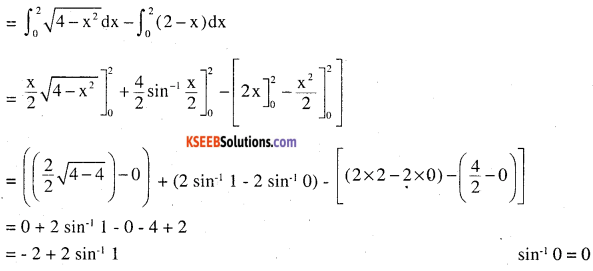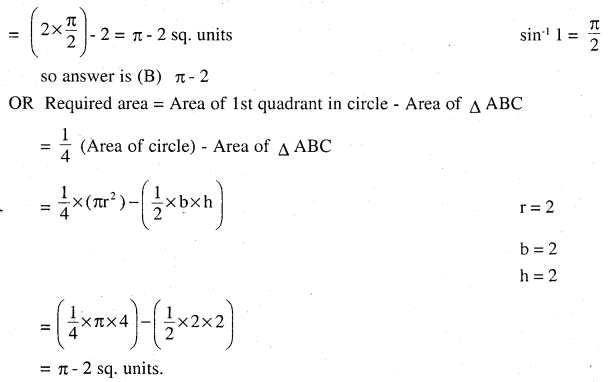# 2nd PUC Maths Question Bank Chapter 8 Application of Integrals Miscellaneous Exercise

Students can Download Maths Chapter 8 Application of Integrals Miscellaneous Exercise Questions and Answers, Notes Pdf, 2nd PUC Maths Question Bank with Answers helps you to revise the complete Karnataka State Board Syllabus and score more marks in your examinations.

## Karnataka 2nd PUC Maths Question Bank Chapter 8 Application of Integrals Miscellaneous Exercise

Question 1.
Find area under gives curves and line:
(i) y = x2, x = I, x = 2 and x – axis
(ii) y = x2, x = 1, x = 5 and x – axis
(i) y = x2 is upward parabola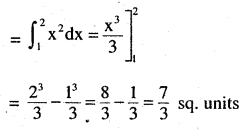(ii) y = x4 is upward parabola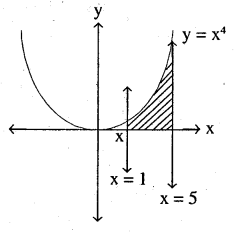Question 2.
Find the area between the curves y = x and y = x2.
y = x is line with pts (0, 0) (-1, 1) (1, 1) (2, 2) etc
y = x2 is parabola with vertex (0,0) up ward parabola
They meet at pts (0, 0) and (1, 1)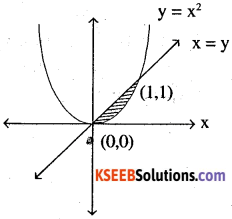⇒ y = x putting in y = x2
x = x2 ⇒ x = 0 ⇒ y = 0
x =1 ⇒ y = 1
Area is (shaded one) = (Area under line x = 0 to x = 1)
– (Area under parabola x = 0 to x = 1)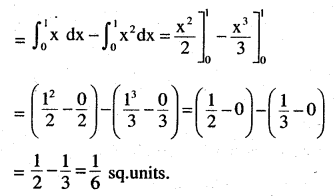Question 3.
Find the area of the region lying in the first quadrant and bounded by
y = 4x2, x = 0, y = 1 and y = 4.
(1) y = 4x2 is an upward parabola with vertex (0, 0)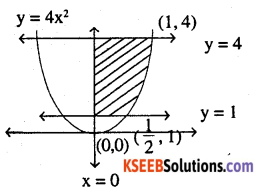x = 0 is line with pts (0,1) (0,0) etc….
y = 1 is line with pts (0, 1) (1, 1) etc….
y = 4 is line with pts (0,4) (1, 4) etc….
parabola meets at pts (0,0) with line x = 0
(1/2,1) with line y = 1 (1,4) with line y = 4
Here we need to do along ‘y’ axis as we don’t know eq: of line with respect to ‘x’. Required area (shaded one) = Area under parabola from y = 1 to y = 4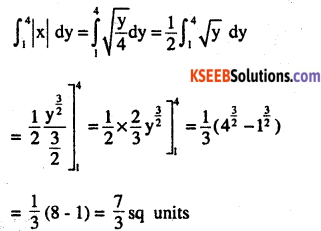Question 4.
Sketch the graph of y = |x+3| and evaluate $$\int _{ -6 }^{ 0 } |x+3|dx$$
y = |x+3|
points are    x = 0, y = 3 ; x = 1, y = 4 etc
x = -1, y = 2 ; x = -4, y = (-1) =   1
so point are (0, 3), (1,4), (2, 5), (-1,2), (-2, 1) (-3, 0), (-4,1) etc.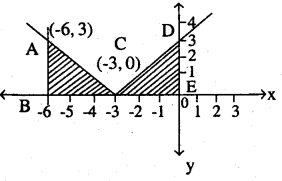Question 5.
Find the area bounded by the curve y = sin x between x = 0 and x = 2π
Sin curve is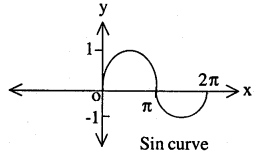y = |sin x| is a sin curve
x = 0 is a line pts (0,1) (0, 2) etc
x = 2 π is line through pts 2 π
Here in this we take n multiples in ‘x’ axis and 1 and -1 in ‘y’ axis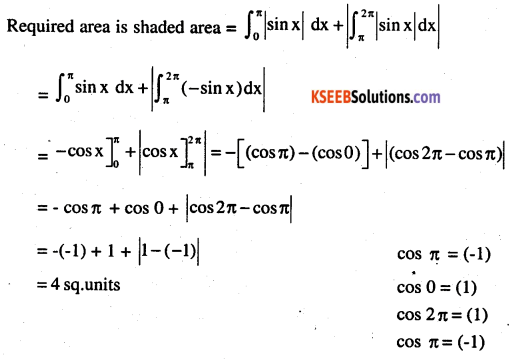Question 6.
Find the area enclosed between the parabola y2 = 4ax and the line y = mx.
y2 = 4ax is right sided parabola vertex (O, O). y
y = mx is straight line through (O, O)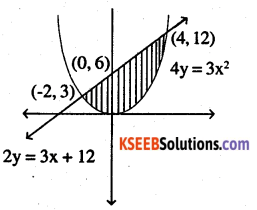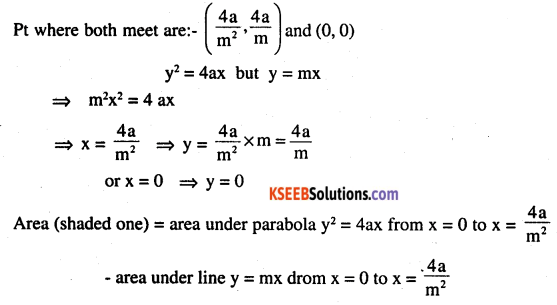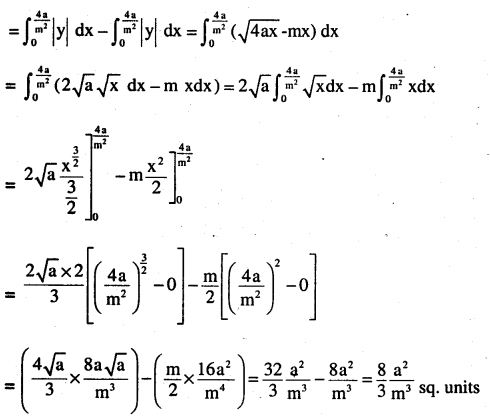Question 7.
Find the area enclosed by the parabola 4y = 3x2 and the line 2y = 3x + 12.
4y = 3x2 is parabola upward
line 2y = 3x +12X 0 2 -2 y 6 0 3

They meet at pts (4,12) and (-2,3)
4y = 3x2
putting 2y = 3x +12 in it
2(3x +12) = 3x2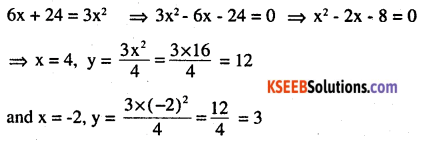Required area (shaded) = Area under line 2y = 3x + 12 from x = -2 to x = 4
– Area under parabola 4y = 3x2 from x = -2 to x = 4Question 8.
Find the area of the smaller region bounded by the ellipse
$$\frac{x^{2}}{9}+\frac{y^{2}}{4}=1 \text { and the line } \frac{x}{3}+\frac{y}{2}=1$$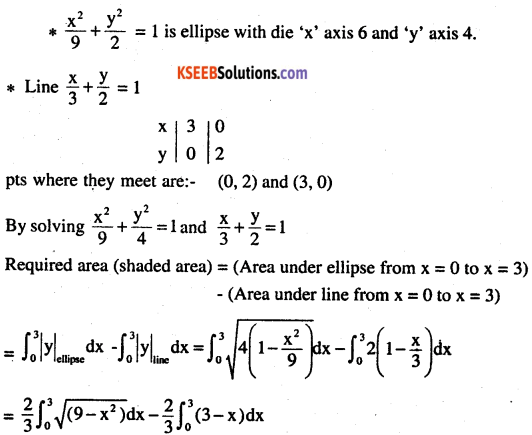Required area (shaded area) = Area under ellipse from x = 0 to x = 3)
-(Area under line from x = 0 to x = 3)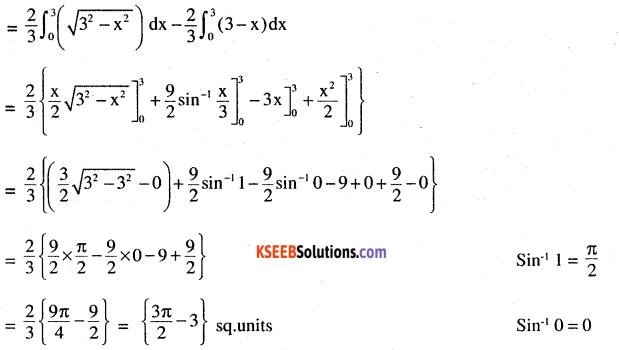Question 9.
Find the area of the smaller region bounded by the ellipse
$$\frac{x^{2}}{a^{2}}+\frac{y^{2}}{b^{2}}=1 \text { and the line } \frac{x}{a}+\frac{y}{b}=1$$Required area (shaded area) = Area under ellipse from x = 0 to x = 3)
-(Area under line from x = 0 to x = 3)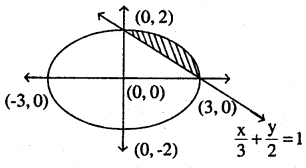OR
Required area (Shaded) = Area of ellipse in 1st quadrant – Area of Δ ABC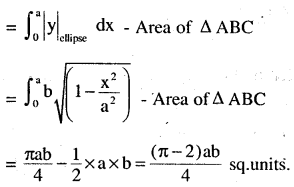Question 10.
Find the area of the region enclosed by the parabola x2 = y, the line y = x + 2 and the
x – axis.
Ans:
x2 = y is an upward parabola
line y = x +2They meet at pts (2, 4) and (-1, 1)
By solving the equation
x2 = y, y = x + 2
⇒ x2– x – 2 = 0
⇒ x = 2 or -1
y = 4 or 1
$$Required\quad area=\int _{ -1 }^{ 2 } Area\quad of\quad line-\int _{ -1 }^{ 2 } Area\quad of\quad parabola$$Question 11.
Using the method of integration find the area bounded by the curve |x| + |y| = 1.
[Hint: The required is bounded by lines x + y = 1, – x + y = 1 and -x -y = 1].
|x|+ |y| = 1so points are (0, 1) (0, -1) (1, 0) (-1, 0)
but |x| + |y| =  1 can be written as: follows: ⇒ -x + y = 1 (2nd quadrant); x + y =1
(1st quadrant); x – y = 1 (4th qudrant); -x -y = 1 (3rd quadrant) .Not by Integration
= 4 x Area of any one part Δ
= 4 x Area Δ ABC
$$=4 \times \frac{1}{2} \times(\mathrm{AB}) \times|\mathrm{BC}|$$
$$=4 \times \frac{1}{2} \times 1 \times 1=2 \mathrm{sq.units.}$$By integration
Area required
= 4 x Area of ΔABC
= 4 x Area under line AC (x + y = 1)Question 12.
Find the area bounded by curves {(x, y): y ≥ x2 and y = |x| }.
y ≥ x2 and y ≤ |x|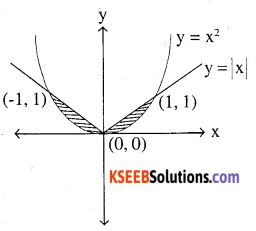consider then as curves y = x2 (upward parabola vertex (0, 0)
y= |x| (line)
As y ≥ x2, so area above parabola
y ≤ |x|, so area below modulus
∴ required area is shaded one
= 2x [Area under line in 1st quadrant)
– (Area under parabola in 1 st quadrant)]Question 13.
Using the method of integration find the area of the triangle ABC, coordinates of whose
vertical are A(2,0), B(4 ,5) and C (6 , 3)
By 2 points form, equation of the line is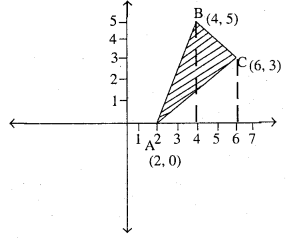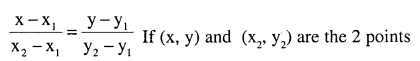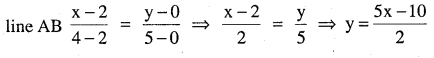Question 14.
Using the method of integration find the area of the region bounded by lines:
2x + y = 4,3x – 2y = 6 and x – 3y + 5 = 0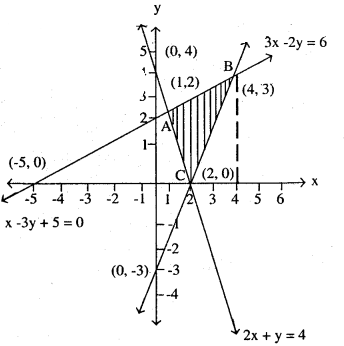Required area (shaded) = Area under line AB – Area under AC -Area under BC
$$=\int_{1}^{4} \text { line } \mathrm{AB}-\int_{1}^{2} \text { line } \mathrm{AC}-\int_{2}^{4} \text { line } \mathrm{BC}$$Question 15.
Find the area of the region {(x, y) : y2< 4x, 4×2 + 4y2< 9}
Curve y2 = 4x is a parabola right sided
Curve 4x2 + 4y2 = 9 is a circle with radius $$\frac{3}{2}$$ and centre (0, 0)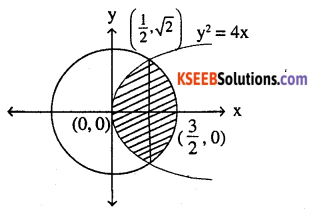They meet at points
$$\left(\frac{1}{2}, \sqrt{2}\right)$$
They meet at points
y2 = 4x in 4x2 + 4y2 = 9
⇒ 4x2 + 4 (4x) = 9
⇒ 4x2+16x – 9 = 0
$$\Rightarrow x=\frac{1}{2}, \frac{-9}{2} \text { but } x \ 0, \text { so } \frac{-9}{2} \text { not possible }$$
So, required area (shaded) = 2 x half of the area we get above ‘x’ axis
= 2 x [Area under curve y2= 4x + Area under curve 4x2 + 4y2 = 9]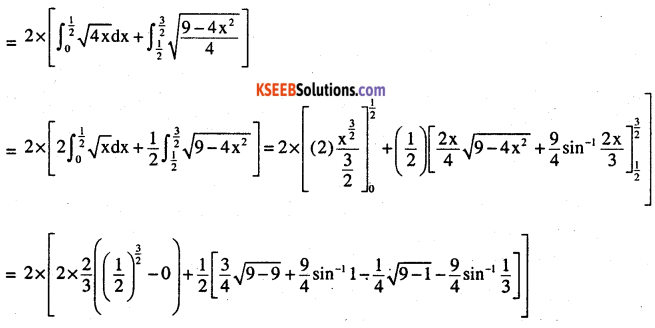Choose the correct answer in the following Exercise from 16 to 20.

Question 16.
Area bounded by the curve y = x3, the x-axis and the ordinates x = -2 and x = 1 is
(A) -9
(B)$$\frac{-15}{4}$$
(C)$$\frac{15}{4}$$
(D)$$\frac{17}{4}$$
y = x3= Area under curve y = x3 with respect to ‘x’ axis from x = -2 to x = 1Question 17.
The area bounded by the curve y = x |x| , x – axis and the ordinates x = -1 and x = 1 is given by
(A) 0
(B)$$\frac{1}{3}$$
(C)$$\frac{2}{3}$$
(D)$$\frac{4}{3}$$
[Hint: y = x2 if x > 0 and y = – x2 if x < 0].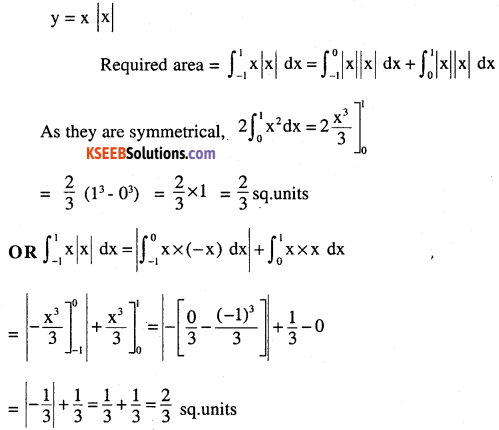NOTE: As explained in summary of this chapter
So correct answer is (C) $$\frac{2}{3}$$

Question 18.
The area of the circle x2 + y2 = 16 exterior to the parabola y2 = 6x is
(A) $$\frac{4}{3}(4 \pi-\sqrt{3})$$
(B) $$\frac{4}{3}(4 \pi+\sqrt{3})$$
(C) $$\frac{4}{3}(8 \pi-\sqrt{3})$$
(D) $$\frac{4}{3}(8 \pi+\sqrt{3})$$x2 + y2 = 16 is circle with radius 4 centre (0, 0)
y2 = 6x is is right side parabola, vertex (0, 0)
They both meet at pts:
x2 + y2 = 16, But given y2 = 6x
so x2 + 6x – 16 = 0 ⇒ x = -8, but x <0 is eliminated
x = 2, $$y=2 \sqrt{3}$$
Required area (shaded) = Area of circle – shaded area (as ext. to parabola in circle)
Shaded area = 2 x [(Area of parabola from x = 0 to x = 2) +
(Area of circle from x = 2 to x = 4)]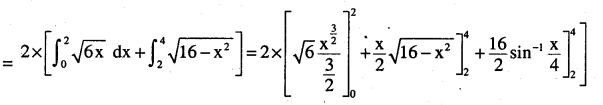Question 19.
The area bounded by the y – axis, y = cos x and y = sin x when $$0 \leq x \leq \frac{\pi}{2} \text { is }$$
(A)$$2(\sqrt{2-1})$$
(B)$$\sqrt{2}-$$
(C)$$\sqrt{2}+1$$
(D)$$\sqrt{2}$$2nd PUC  Maths Application of Integrals Miscellaneous Exercise Additional Questions and Answers

Question 1.
Find the area of ABC, where vertices are A (4, 1) B (6, 6), C (8, 4) (CBSE 2010)Question 2.
Find the area of the circle 4x2 + 4y2 = 9, which is interior to the parabola x2 = 4y.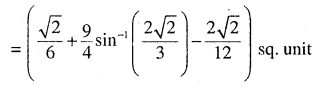Question 3.
Sketch the graph of y=|x+3| and evaluate x-axis and between x = – 6 and x = 0.
(CBSE – 2011)Question 4.
Find the area of the region {(x, y) : x2 + y2 = 4, x + y ≥ 2}  (CBSE 2012)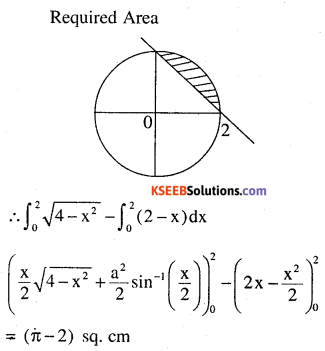Circle x2 + y2 = 4 his centre (0, 0) and radius 2
line x + y = 2 pts are (1,1) (2, 0) (0, 2) etc………
circle and line intersect at pts.
It can be found by solving the equation
x2 + y2 = 4 put (y = 2 – x)
⇒ x2 + (2 -x)2 = 4 ⇒ 2x2 – 4x + 0 = 0
⇒ 2x2 – 4x = 0 ⇒ x2 – 2x = 0
⇒ (x -2) x = 0  ⇒ x = 0 or x = 2
so y = 2 – x , x = 0 , y = 2    (0, 2)
x = 2 , y = 0 , (2,0)
so they meet at (0, 2) and (2, 0)